I wanted a system to detect the passage of vehicule that is cheap and rapidly deployable. A microphone was used as sensor because the sound of a vehicle is very recognizable. Of course there are others ways available to detect a vehicle (pneumatic road tube, piezoelectric sensor, inductive loop, magnetic sensor, microwave sensor, infrared sensor, cameras) but a microphone is very cheap, can be found in mass market, is very fast to install and contrary to a camera, it works at night.
System setup
The recording is done via a single microphone connected to a Raspberry Pi. The electric current is supplied to the Raspberry Pi by an external USB battery. The running time depends of the capacity of the battery, in the current setup, it took 4 hours to empty the battery with the Raspberry Pi’s CPU running at full speed. The microphone is a Trust Micro USB microphone which come with an USB sound card. This external sound card is necessary because the Raspberry Pi’s sound card hasn't any jack input. In this experiment, the system is connected with WiFi and is remotely connected with ssh. To protect the system in rain conditions, an upside down box open on one side permit the microphone to capture the sound and protect it from water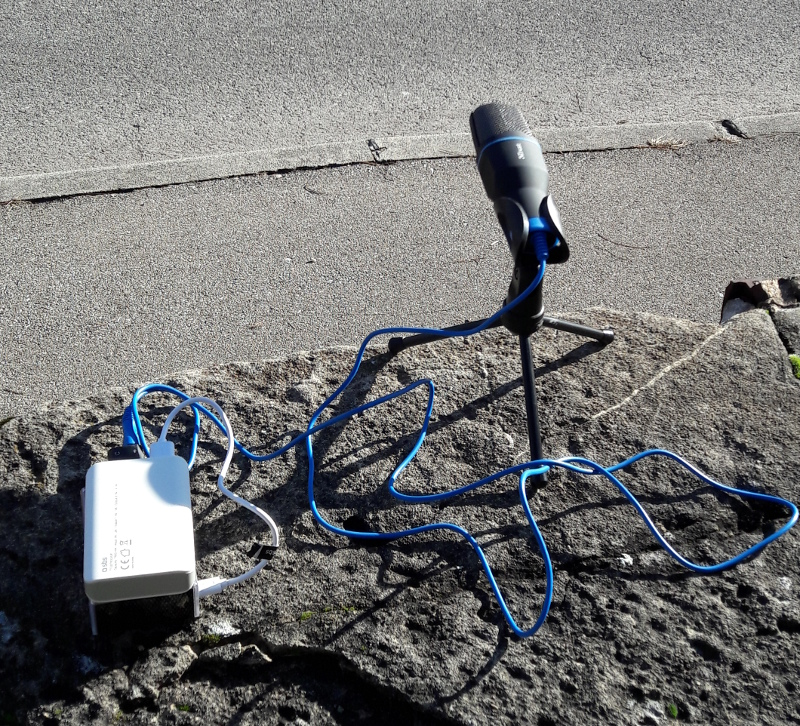Figure 1: Installed setup.

Audio profile
Wavelet transform on recorded audio, shows that the background noise like winds is mainly lowfrequencies while the passage of a vehicle generate harmonics and high frequencies. The wavelettransform were computed using Morlet-Wavelet between 16Hz and 2000Hz on a 4 seconds window. The figure 2 shows the wavelet transform when a vehicle pass in front of the microphone and the figure 3 shows the wavelet transform of the background only.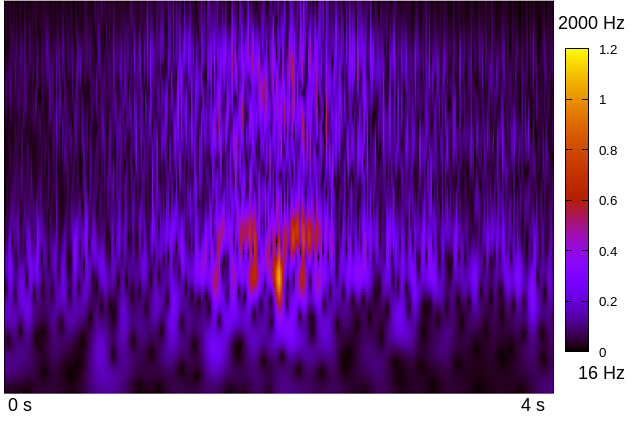Figure 2: Wavelet transform (vehicle passing)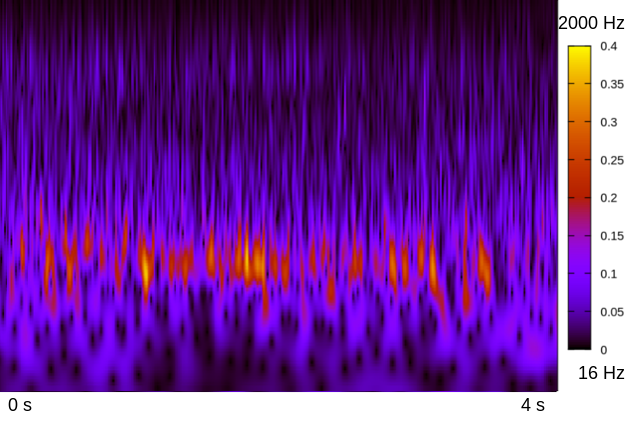Figure 3: Wavelet transform (noise)

Vehicle detection
Each 500 ms, the system analyze a 44100Hz audio signal on a 4 seconds window. The first step of the signal processing consist in a fast analysis to determines if the signal worth being analyzed further to find a vehicle. This is done by dividing the signal in 500 samples then by computing the sound power 25 samples by 25 samples. By experimenting, it was found that the power above a threshold of 0.0001 (dimensionless value that comes from the microphone) is enough to select all passing vehicle. (The amplitude measured is comprise between -1 and 1, respectively the minimum and maximal amplitude measurable by the microphone). If that prerequisite test is passed, the full 4 second signal is processed by another function to analyze it further. To determine if there is a vehicle during these 4 seconds of recorded sound, the first step is to remove the noise. Assuming that the noise is stationary, it is removed from the signal $S(\omega)$ by using a Wiener filter $W(\omega)$ with a pre-recorded signal when a vehicle pass just in front of the microphone $V(\omega)$ and a pre-recorded signal of the noise $N(\omega)$. This filter is applied to the signal $S(\omega)$ to obtains the signal $\tilde S(\omega)$ $$W(\omega) = \frac{V(\omega)}{V(\omega) + N(\omega)}$$ $$\tilde {S}(\omega) = W(\omega) \cdot S(\omega)$$ Once denoised, the signal is cut in many windows of 1000 samples each. Then for each window, an FFT is applied. To avoid artifacts by doing the FFT directly on a squared window, a Hamming window is first computed to avoid brutal change at the border. For each FFT, the median between the lowest and the highest frequency whose amplitude is above a threshold is stored in an array. This gives the variation of the median frequency in the signal. The result is then smoothed with a Gaussian. When a vehicle pass in front of the microphone, there is a high peak of median frequency. To detect these peaks, the software detects the sign changes in derivative to determines if it’s a peak or not and select only the 3 highest peaks in the window.
In the figure 4, the big peaks over 1400Hz corresponds to the passage of a vehicle, the 4 peaks at 70s, 80s and 170s and 220s are also the result from a passage of a vehicle but than can be hear from a near roads.
Another used property is the power of the signal $x$ when a vehicle pass in front of the micro-phone. For each windows of 1000 samples, the power is computed using: $$\frac{1}{1000} \sum_{i=0}^{1000} x[i]^2$$ When a vehicle pass in front of the microphone there is a clear peak as show in figure 5.
The power alone is not sufficient to determine if there is a vehicle or not (there are big peaks at 70s and 250s even if there is no vehicle), but it can be used to determines the power threshold from where a vehicle can be found.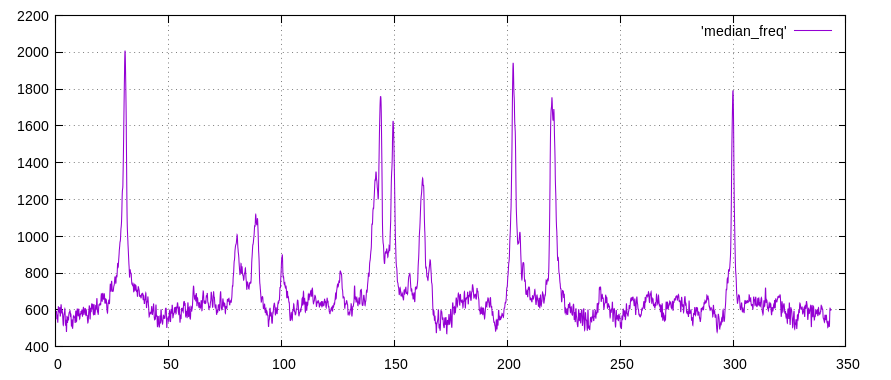Figure 4: Computed median frequency over time of a 350s record using a window of 1000samples.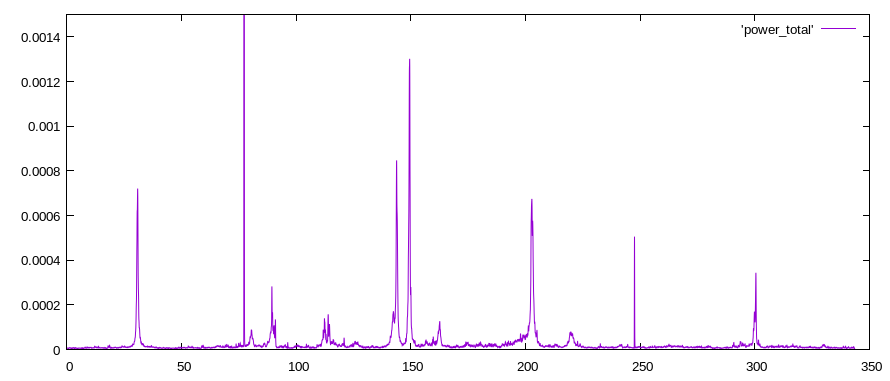Figure 5: Computed power of a 350s record (dimensionless measure from mic).

The same computations are done with a reference signal of a passing vehicle and the similarity is computed by the cross-correlation of the median frequency and the power with the following formula. $$\max_n \frac{\sum_i s_1[i+n] \cdot s_2[i]}{\sum_i s_2[i]^2}$$ The correlation of the signal $s_1$ with the reference signal $s_2$ with the offset $n$ that give the best result is keep. Note that in the implementation it look for the best correlation around the peaks of power.
The combined results of the correlation of power over time, the correlation of median frequency over time and the peak value of the median frequency gives a very good ways to determine if there is a vehicle or not.
Results
The dispositif was tested in 3 different places with different wind conditions. Because wind, and the majority of the encountered sources of noise are found in frequencies lower than the important signal, they don’t influence much the result. In rain conditions, the signal is more noisy, but the quality of the signal is still enough good so the system still works accurately (also, the hardware needs to be protected from water with a box). If the system is running near another road, it may count also vehicles on the other road if they can be hear sufficiently loud. The following table shows the results and error rate.
All combined Vehicle No Vehicle
Detected 40 4
Not Detected 6 n.d.